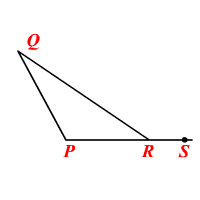# Exterior Angle Inequality

An exterior angle of a triangle is greater than either of the non-adjacent interior angles.In the figure, $\angle QRS$ is an exterior angle.

So, $m\angle QRS>m\angle P$ and $m\angle QRS>m\angle Q$ .